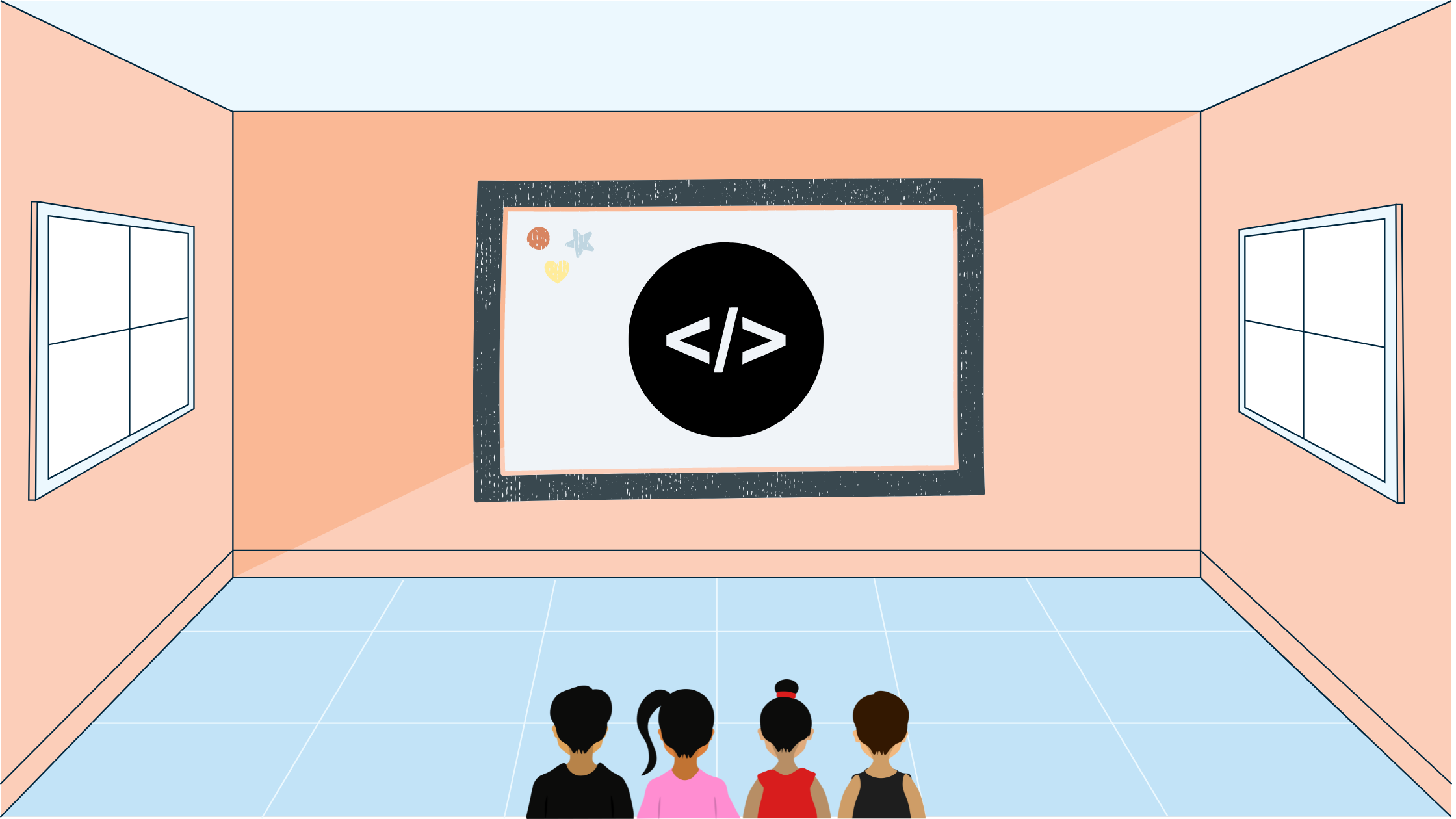Yash Singh# USACO Photoshoot Bronze US Open 2022 Problem 1

In this blog post I'll go over the USACO Photoshoot Bronze US Open 2022 Problem 1 using other resources and solutions.

## Statement

According to the problem statement, the input is a string that consists of two characters, G and H, both representing two separate breeds of cows. The farmer wants to arrange the string in such a manner that as many as possible of the even positions contain a G. The commands he gives to the cows is a number $k$ where the first $k$ cows have to reverse themselves in order.

## Solution

First of all, we can group the cows into pairs because reversals might change a GH to an HG but the two cows will still be in a pair. Next, let's represent all GH's as a 1 and all HG's as a 0. Also represent all GGs and HHs as _s. For example, the following input: GG|HG|GH will become _01. We want to maximize the amount of HG or 0s. Whenever we reverse any prefix, we are flipping the 1s to 0s and 0s to 1s and reversing the order. For example, an operation on a prefix of 5 to the following:

101001_1
110101_1

For clarity, let's drop all _s as they do not have any effect on the end result. Now we have the following: 1101011. Given any string like this, we can come up with an all 0 solution to it, through the following:

1101011
0001011 # flip prefix of 2
1111011 # flip prefix of 3
0000011 # flip prefix of 4
1111111 # flip prefix of 5
0000000 # flip prefix of 7

To get to this point, it took us 5 operations. Notice that in each operation we are "equalizing" the first two segments of opposite bits. For example, when we have a 110 we are turning it into a 000 so we can flip the first three as a whole. We continue this process till all the segments are equalized with one operation for each segment. Let's try another input 010100110.

010100110
110100110 # flip prefix of 1
000100110 # flip prefix of 2
111100110 # flip prefix of 3
000000110 # flip prefix of 4
111111110 # flip prefix of 6
000000000 # flip prefix of 8

Notice how even though we could divide the 010100110 into 7 segments, we took 6 operations since the last segment consisted of 0 which could be neglected. This is an edge case we need to account for in our implementation.

## Implementation

Let's go through an implementation in C++:

#include <bits/stdc++.h>

using namespace std;

int main() {
ios_base::sync_with_stdio(false);
cin.tie(nullptr);

int n;
cin >> n;
int last{-1}, ans{0};
for (int i{0}; i < n; i += 2) {
char x, y;
cin >> x >> y;
// Prevent _s
if (x != y) {
// If the pair is GH (incorrect)
if (x == 'G') {
// If the last pair wasn't GH then we have to flip (otherwise we can group)
if (last != 1) {
++ans;
}
// Set last to GH
last = 1;
}
// Otherwise if the pair is HG (correct)
else { // x == 'H'
// If the last pair wasn't HG
if (last != 0) {
++ans;
}
// Set last to HG
last = 0;
}
}
}
// Handle edge case where last one is already right
// We don't need to flip the last one another time
if (last == 0) {
--ans;
}
cout << ans;
}

## Lessons Learnt

A few lessons learnt through this problem is that you should try to break down and make the input simpler, because through that patterns may emerge that can ultimately lead to the solution.